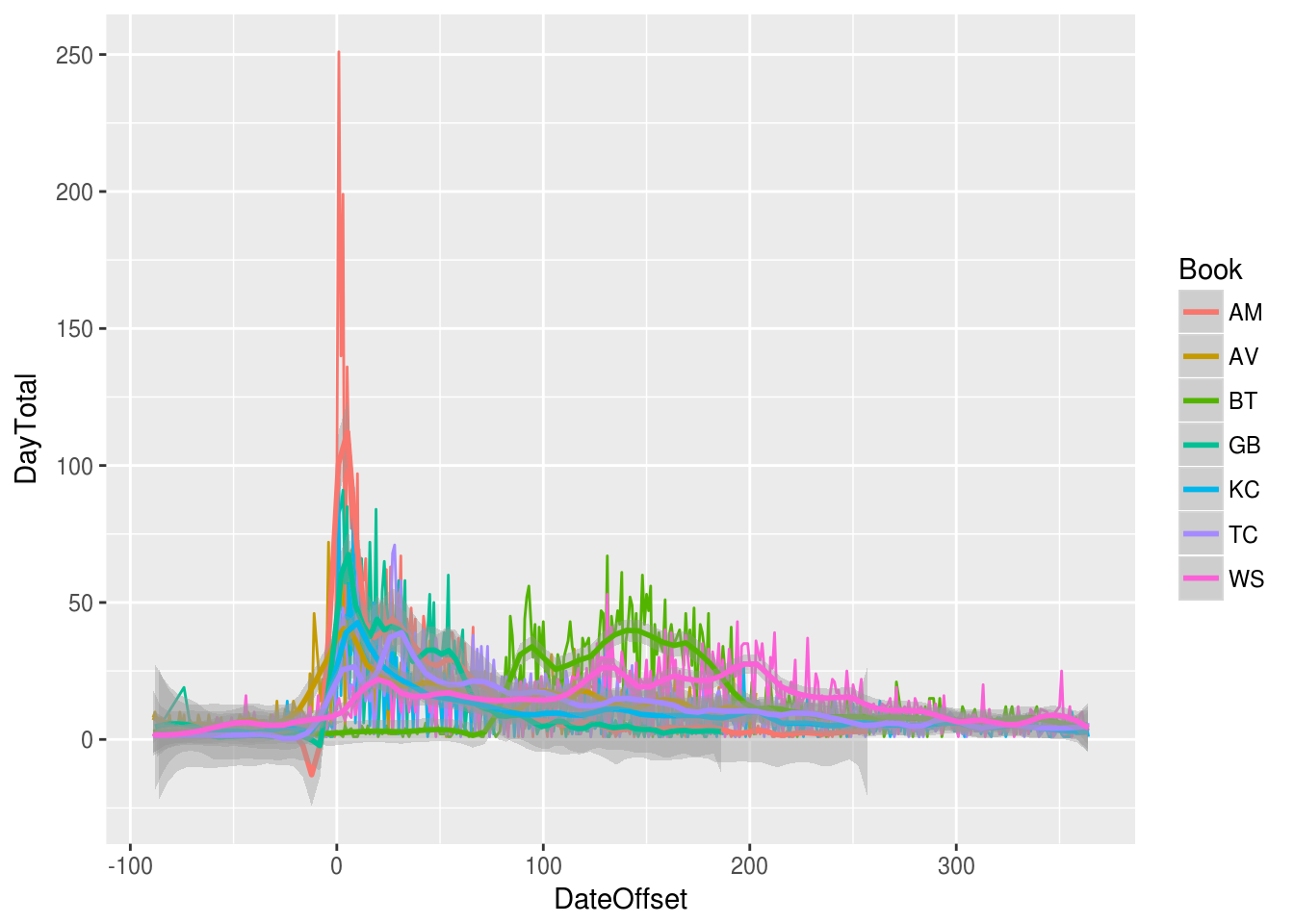This file displays time series visualizations of circulation

``````library(knitr)
setwd("/home/rburke/oboc/src/rcr-analysis/src/viz")

### Project constants

``````# Load project constants
setwd("/home/rburke/oboc/src/rcr-analysis/src/")
source("common.R")``````

``````library("ggplot2")
library("dplyr")
library("RMySQL")``````

### Database connection

``````# Database connection
con <- dbConnect(MySQL(),
dbname="oboc", host="localhost")``````

### Database query

``````# Aggregate by day and book
series_query <- paste("select count(T.id_item), T.abbrev_season, T.day_season ",
"from V_norm_trans2 T ",
"where T.day_season > -90 and T.day_season < 365 ",
"group by T.abbrev_season, T.day_season", sep="")
daily.df <- dbGetQuery(con, series_query)
#daily.df <- dbFetch(rs, n=-1)
colnames(daily.df) <- c("DayTotal", "Book", "DateOffset")
daily.df\$Book <- as.factor(daily.df\$Book)``````

### Daily circulation

``````# Daily line plot
p <- ggplot(data=daily.df, aes(x=DateOffset, y=DayTotal, color=Book))
p <- p + geom_line() + geom_smooth(method="loess", span=0.1)
print (p)``````### Daily circulation smoothed

``````# Just the smoothed line
p <- ggplot(data=daily.df, aes(x=DateOffset, y=DayTotal, color=Book))
p <- p + geom_smooth(method="loess", span=0.1, se=FALSE)
print (p)``````